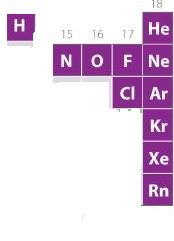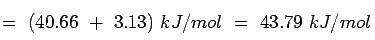Courses

# Test: Effect Of Heat On Matter

## 20 Questions MCQ Test Chemistry Class 11 | Test: Effect Of Heat On Matter

Description
This mock test of Test: Effect Of Heat On Matter for JEE helps you for every JEE entrance exam. This contains 20 Multiple Choice Questions for JEE Test: Effect Of Heat On Matter (mcq) to study with solutions a complete question bank. The solved questions answers in this Test: Effect Of Heat On Matter quiz give you a good mix of easy questions and tough questions. JEE students definitely take this Test: Effect Of Heat On Matter exercise for a better result in the exam. You can find other Test: Effect Of Heat On Matter extra questions, long questions & short questions for JEE on EduRev as well by searching above.
QUESTION: 1

### Among the following sentences the one that is false about gases is

Solution:

Gasses are highly compressible because it is less dense than other states of matter. it's intermolecular force of attraction is less and it has more space between the molecules. Therefore, it is easy to compress gas.
While, solids are almost incompressible. This is because the intermolecular force of attraction in solids is greater compared to other states of matter. The moles in solids are tightly packed. Therefore, solids are almost incompressible.

QUESTION: 2

### A cylinder of V litre capacity contains ammonia gas. This cylinder is inverted over another vessel of V litre capacity containing hydrogen chloride at the same temperature and pressure. The pressure in the cylinder after sometime will

Solution:

As per Gay Lussac’s Law, when pressure and temperature are same V directly proportional to the moles. Let n moles of NH3 and n moles HCl after some time they react and form n moles NH4Cl and hence pressure drops.

QUESTION: 3

### The number of elements that exists in gaseous state under normal atmospheric conditions is

Solution:

A look at the periodic table shows us that there are 11 elements in the table that exist in the gaseous state at room temperature. These elements are Hydrogen, Helium, Nitrogen, Oxygen, Fluorine,  Chlorine, Neon, Argon, Krypton, Xenon, and Radon.QUESTION: 4

The relation between Celsius scale and Fahrenheit scale of measuring temperature is

Solution:

Invented in 1742 by the Swedish astronomer Anders Celsius, it is sometimes called the centigrade scale because of the 100-degree interval between the defined points. The following formula can be used to convert a temperature from its representation on the Fahrenheit ( F) scale to the Celsius (C) value: C = 5/9(F - 32).

QUESTION: 5

Which one will have higher value of enthalpy of vaporization:

Solution:

Higher value of enthalpy of vaporization means that we need to give high heat for the given liquid to vapour. So this is possible with only option b because with only option b, there is enough strong attractive force which we need to overcome by providing the most amount of heat in all the given cases.

QUESTION: 6

The zero point temperature is:

Solution:

It is obvious that a lower temperature is not feasible because there is no velocity smaller than zero and no energy content less than nothing. (As a side remark, energy in this instance means only the energy that can be taken away from the particles and does not include the rest mass or quantum mechanical zero-point energies for confined particles.) Absolute zero corresponds to –273 degrees Celsius and –460 degrees Fahrenheit.

QUESTION: 7

Choose the characteristic property of gases from the following

Solution:

Gases are not at all ridig as compared to solids and liquids  their intre particle arrangement are very far from each other. they do not have definet shape, size & volume.

QUESTION: 8

Choose the one which is not a characteristic property of gases:

Solution:

The Properties of Gases. Gases have three characteristic properties:
(1) they are easy to compress,
(2) they expand to fill their containers, and
(3) they occupy far more space than the liquids or solids from which they form.

QUESTION: 9

Which of the following sublimes at ordinary temperature:

Solution:

Camphor sublimes at ordinary temperature. It's a simple fact.

QUESTION: 10

Thermal energy is responsible for:

Solution:

Thermal energy refers to the energy contained within a system that is responsible for its temperature. Heat is the flow of thermal energy.Heat energy is the result of the movement of tiny particles called atoms,molecules or ions in solids, liquids and gases. Heat energy can be transferred from one object to another. The transfer or flow due to the difference in temperature between the two objects is called heat.

QUESTION: 11

Thermal energy is directly proportional to:

Solution:

Thermal energy is the sum of all the random kinetic energies of the molecules in a substance, that is, the energy in their motions. The higher the temperature, the greater the thermal energy. On the Kelvin temperature scale, thermal energy is directly proportional to temperature.

QUESTION: 12

The conditions necessary for a gas to liquefy are

Solution:

Liquiefing gas means changing of the substances in gaseous states are changed into liquid state.

To liquefy gas we must-

- Decrease temperature
- Increase pressure

By doing this the molecules of gas will come closer to each other and temperature is reduced so it will remove the enough energy to convert gas into liquid state. Thomas Andrews did work on liquefaction of gases like carbon dioxide.

QUESTION: 13

The temperature in Celsius scale can be converted into Kelvin scale

Solution:

To convert from Celsius to Kelvin you use the following formula:

Celsius temperature + 273.15 = Kelvin Temperature

For example:
26 °Celsius + 273.15 = 299.15 Kelvin

The Kelvin temperature scale was designed so that it starts at absolute zero. In Kelvin, absolute zero is equal to 0 degrees. In Celsius, absolute zero is equal to −273.15 degrees.

Therefore, you need to add 273.15 to the Celsius temperature to get to the Kelvin temperature.

Note: Kelvin does not use the degree symbol, °.

QUESTION: 14

What happens to density of liquids on increasing temperatures:

Solution:

Density increase as the temperature decreases. This is the reason why liquid water is more dense than solid water. The bonds in water break more slowly as temperature decreases and the structure tend to trap fewer extra water molecules. At low temperature, more of the water has the same lattice as ice.

QUESTION: 15

What happens to boiling point of a liquid when pressure is increased:

Solution:

The Boiling point of a liquid is the temperature at which the vapour pressure of the liquid equals the external pressure.

Also the vapour pressure of a liquid is a function of the temperature. As the temperature increases, the vapour pressure increases.

QUESTION: 16

Sublimation involves the direct state change from:

Solution:

Sublimation or volatilization is a change of state from solid to gas without passing through the liquid state. A typical example is dry ice, which can sublimate at r.t. and at atmospheric pressure.

QUESTION: 17

Standard enthalpy of vaporization is taken at:

Solution:

The standard enthalpy of vaporization of water at 298 K and 1 bar is thus:QUESTION: 18

The standard boiling point of the liquid is

Solution:

The standard boiling point is defined as the temperature at which boiling takes place under a pressure of 1 bar.

Whereas, the normal boiling point is defined as the temperature at which the vapour pressure of the liquid is equal to 1 atm at sea level.

Since 1 atm is equal to 1.01325 bar and hence is greater than 1 bar, the normal boiling point temperature is greater than the standard boiling point temperature.

QUESTION: 19

Normal boiling point is

Solution:

The boiling point of a liquid at 1 atm is also known as the normal boiling point. It has been found that Helium has the lowest normal boiling point (−268.9 °C) because it has very weak intermolecular attractions. Liquids that have high vapour pressures and low boiling points are known to be volatile, which means they evaporate quickly at room temperature. When the temperature increases, molecules start entering the gaseous state, consequently increasing the vapor pressure of the liquid until it comes into equilibrium with the atmospheric pressure.

QUESTION: 20

In the process of conversion of a solid to liquid the average distance between the molecules is

Solution:

The correct answer is option B
In ordered solids, the atomic spacing between two bonded atoms is generally around a few ångströms (Å), which is on the order of 10−10 meters. However, in very low density gasses (for example, in outer space) the average distance between atoms can be as large as a meter.